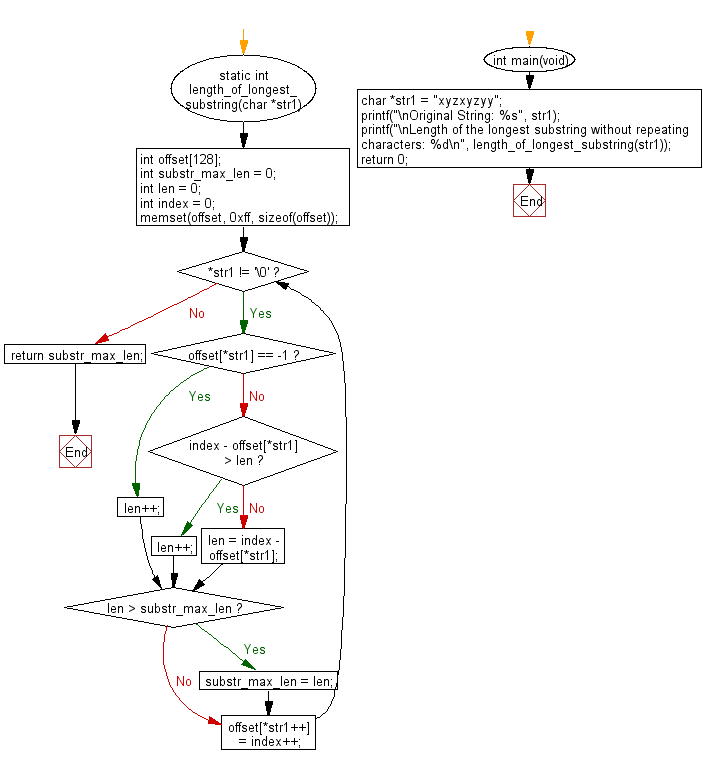﻿ C Program: No repeating characters in a substring# C Exercises: Find length of the longest substring of a given string without repeating characters

## C Programming Practice: Exercise-2 with Solution

Write a C program to find length of the longest substring of a given string without repeating characters.

Note:
1) Given string consists of English letters, digits, symbols and spaces.
2) 0 <= Given string length <= 5 * 104

Difficulty: Medium.

Input String : xyzxyzyy
The longest Substring Length : 3
The longest substring : xyz

Input String : pickoutthelongestsubstring
The longest Substring Length : 8
The longest substring : ubstring

Input String : ppppppppppppp
The longest substring : p
The longest Substring Length : 1

Input String : Microsoft
The longest substring : Micros
The longest Substring Length : 6

C Code:

``````#include <stdio.h>
#include <stdlib.h>
#include <string.h>
static int length_of_longest_substring(char *str1)
{
int offset;
int substr_max_len = 0;
int len = 0;
int index = 0;
memset(offset, 0xff, sizeof(offset));
while (*str1 != '\0') {
if (offset[*str1] == -1) {
len++;
}
else {
if (index - offset[*str1] > len)
{
len++;
}
else
{
len = index - offset[*str1];
}
}
if (len > substr_max_len)
{
substr_max_len = len;
}
offset[*str1++] = index++;
}
return substr_max_len;
}
int main(void)
{
char *str1 = "xyzxyzyy";
printf("\nOriginal String: %s", str1);
printf("\nLength of the longest substring without repeating characters: %d\n", length_of_longest_substring(str1));
return 0;
}
``````

Sample Output:

```Original String: xyzxyzyy
Length of the longest substring without repeating characters: 3
```

Pictorial Presentation:Flowchart:## C Programming Code Editor:

What is the difficulty level of this exercise?

Test your Programming skills with w3resource's quiz.

﻿

## C Programming: Tips of the Day

Maximum value of int:

In C:

```#include <limits.h>
then use
int imin = INT_MIN; // minimum value
int imax = INT_MAX;```

or

```#include <float.h>

float fmin = FLT_MIN;  // minimum positive value
double dmin = DBL_MIN; // minimum positive value

float fmax = FLT_MAX;
double dmax = DBL_MAX;```

Ref : https://bit.ly/3fi8yk9